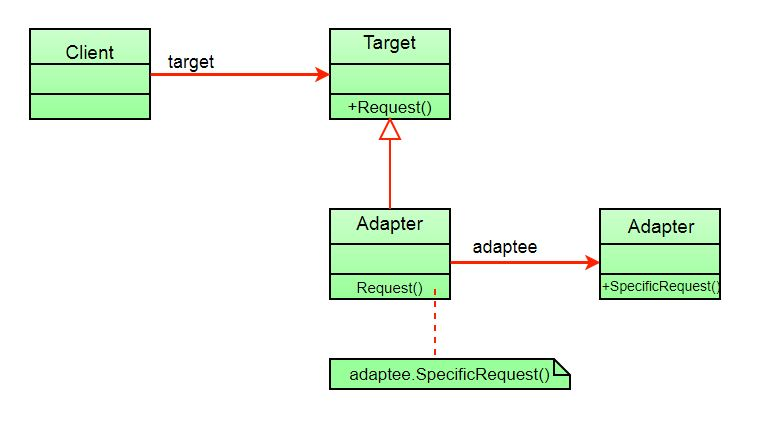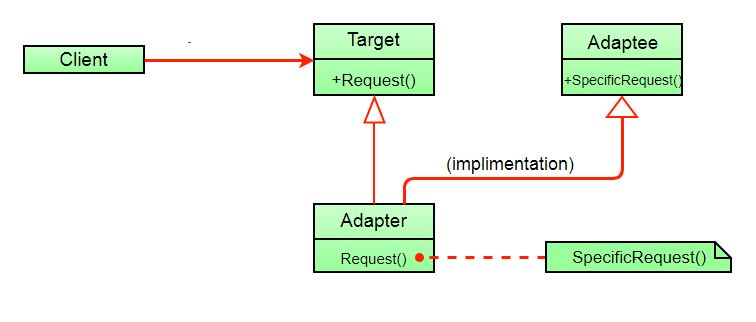Open in App
Not now

• Difficulty Level : Medium
• Last Updated : 27 Jan, 2023

This pattern is easy to understand as the real world is full of adapters.   For example consider a USB to Ethernet adapter. We need this when we have an Ethernet interface on one end and USB on the other. Since they are incompatible with each other. we use an adapter that converts one to other. This example is pretty analogous to Object Oriented Adapters. In design, adapters are used when we have a class (Client) expecting some type of object and we have an object (Adaptee) offering the same features but exposing a different interface.

1. The client makes a request to the adapter by calling a method on it using the target interface.
3. Client receive the results of the call and is unaware of adapter’s presence.

Definition: The adapter pattern convert the interface of a class into another interface clients expect. Adapter lets classes work together that couldn’t otherwise because of incompatible interfaces. Class Diagram:The client sees only the target interface and not the adapter. The adapter implements the target interface. Adapter delegates all requests to Adaptee. Example:

Suppose you have a Bird class with fly() , and makeSound()methods. And also a ToyDuck class with squeak() method. Let’s assume that you are short on ToyDuck objects and you would like to use Bird objects in their place. Birds have some similar functionality but implement a different interface, so we can’t use them directly. So we will use adapter pattern. Here our client would be ToyDuck and adaptee would be Bird.

Below is Java implementation of it.

## Java

 `// Java implementation of Adapter pattern` `interface` `Bird``{``    ``// birds implement Bird interface that allows``    ``// them to fly and make sounds adaptee interface``    ``public` `void` `fly();``    ``public` `void` `makeSound();``}` `class` `Sparrow ``implements` `Bird``{``    ``// a concrete implementation of bird``    ``public` `void` `fly()``    ``{``        ``System.out.println(``"Flying"``);``    ``}``    ``public` `void` `makeSound()``    ``{``        ``System.out.println(``"Chirp Chirp"``);``    ``}``}` `interface` `ToyDuck``{``    ``// target interface``    ``// toyducks dont fly they just make``    ``// squeaking sound``    ``public` `void` `squeak();``}` `class` `PlasticToyDuck ``implements` `ToyDuck``{``    ``public` `void` `squeak()``    ``{``        ``System.out.println(``"Squeak"``);``    ``}``}` `class` `BirdAdapter ``implements` `ToyDuck``{``    ``// You need to implement the interface your``    ``// client expects to use.``    ``Bird bird;``    ``public` `BirdAdapter(Bird bird)``    ``{``        ``// we need reference to the object we``        ``// are adapting``        ``this``.bird = bird;``    ``}` `    ``public` `void` `squeak()``    ``{``        ``// translate the methods appropriately``        ``bird.makeSound();``    ``}``}` `class` `Main``{``    ``public` `static` `void` `main(String args[])``    ``{``        ``Sparrow sparrow = ``new` `Sparrow();``        ``ToyDuck toyDuck = ``new` `PlasticToyDuck();` `        ``// Wrap a bird in a birdAdapter so that it``        ``// behaves like toy duck``        ``ToyDuck birdAdapter = ``new` `BirdAdapter(sparrow);` `        ``System.out.println(``"Sparrow..."``);``        ``sparrow.fly();``        ``sparrow.makeSound();` `        ``System.out.println(``"ToyDuck..."``);``        ``toyDuck.squeak();` `        ``// toy duck behaving like a bird``        ``System.out.println(``"BirdAdapter..."``);``        ``birdAdapter.squeak();``    ``}``}`

## C++

 `// Java implementation of Adapter pattern``#include ` `class` `Bird``{``  ``public``:``    ``virtual` `void` `fly() = 0;``    ``virtual` `void` `makeSound() = 0;``};` `class` `Sparrow : ``public` `Bird``{``  ``public``:``    ``void` `fly() override``    ``{``        ``std::cout << ``"Flying"` `<< std::endl;``    ``}``    ``void` `makeSound() override``    ``{``        ``std::cout << ``"Chirp Chirp"` `<< std::endl;``    ``}``};` `class` `ToyDuck``{``  ``public``:``    ``virtual` `void` `squeak() = 0;``};` `class` `PlasticToyDuck : ``public` `ToyDuck``{``  ``public``:``    ``void` `squeak() override``    ``{``        ``std::cout << ``"Squeak"` `<< std::endl;``    ``}``};` `class` `BirdAdapter : ``public` `ToyDuck``{``  ``public``:``    ``BirdAdapter(Bird &bird) : bird_(bird) {}``    ``void` `squeak() override``    ``{``        ``bird_.makeSound();``    ``}` `  ``private``:``    ``Bird &bird_;``};` `int` `main()``{``    ``Sparrow sparrow;``    ``PlasticToyDuck toyDuck;` `    ``ToyDuck &birdAdapter = *(``new` `BirdAdapter(sparrow));` `    ``std::cout << ``"Sparrow..."` `<< std::endl;``    ``sparrow.fly();``    ``sparrow.makeSound();` `    ``std::cout << ``"ToyDuck..."` `<< std::endl;``    ``toyDuck.squeak();` `    ``std::cout << ``"BirdAdapter..."` `<< std::endl;``    ``birdAdapter.squeak();` `    ``return` `0;``}``// This code is contributed by Ahmed Izz Murtaja`

## C#

 `using` `System;` `interface` `Bird``{``    ``void` `fly();``    ``void` `makeSound();``}` `class` `Sparrow : Bird``{``    ``public` `void` `fly()``    ``{``        ``Console.WriteLine(``"Flying"``);``    ``}``    ``public` `void` `makeSound()``    ``{``        ``Console.WriteLine(``"Chirp Chirp"``);``    ``}``}` `interface` `ToyDuck``{``    ``void` `squeak();``}` `class` `PlasticToyDuck : ToyDuck``{``    ``public` `void` `squeak()``    ``{``        ``Console.WriteLine(``"Squeak"``);``    ``}``}` `class` `BirdAdapter : ToyDuck``{``    ``Bird bird;``    ``public` `BirdAdapter(Bird bird)``    ``{``        ``this``.bird = bird;``    ``}` `    ``public` `void` `squeak()``    ``{``        ``bird.makeSound();``    ``}``}` `class` `MainClass``{``    ``public` `static` `void` `Main (``string``[] args)``    ``{``        ``Sparrow sparrow = ``new` `Sparrow();``        ``ToyDuck toyDuck = ``new` `PlasticToyDuck();` `        ``ToyDuck birdAdapter = ``new` `BirdAdapter(sparrow);` `        ``Console.WriteLine(``"Sparrow..."``);``        ``sparrow.fly();``        ``sparrow.makeSound();` `        ``Console.WriteLine(``"ToyDuck..."``);``        ``toyDuck.squeak();` `        ``Console.WriteLine(``"BirdAdapter..."``);``        ``birdAdapter.squeak();``    ``}``}`

Output:

```Sparrow...
Flying
Chirp Chirp
ToyDuck...
Squeak
Chirp Chirp```

Explanation : Suppose we have a bird that can makeSound(), and we have a plastic toy duck that can squeak(). Now suppose our client changes the requirement and he wants the toyDuck to makeSound than ? Simple solution is that we will just change the implementation class to the new adapter class and tell the client to pass the instance of the bird(which wants to squeak()) to that class. Before : ToyDuck toyDuck = new PlasticToyDuck(); After : ToyDuck toyDuck = new BirdAdapter(sparrow); You can see that by changing just one line the toyDuck can now do Chirp Chirp !! Object Adapter Vs Class Adapter The adapter pattern we have implemented above is called Object Adapter Pattern because the adapter holds an instance of adaptee. There is also another type called Class Adapter Pattern which use inheritance instead of composition but you require multiple inheritance to implement it. Class diagram of Class Adapter Pattern:Here instead of having an adaptee object inside adapter (composition) to make use of its functionality adapter inherits the adaptee. Since multiple inheritance is not supported by many languages including java and is associated with many problems we have not shown implementation using class adapter pattern. Advantages:

• Helps achieve reusability and flexibility.
• Client class is not complicated by having to use a different interface and can use polymorphism to swap between different implementations of adapters.

• All requests are forwarded, so there is a slight increase in the overhead.
• Sometimes many adaptations are required along an adapter chain to reach the type which is required.